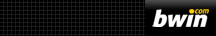### Even oddsThe terms “even odds”, “even money” or simply “evens” (1 to 1, or 2 for 1) imply that the payout will be one unit per unit wagered plus the original stake, that is, ‘double-your-money’. Assuming there is no bookmaker fee or built-in profit margin, the actual probability of winning is 50%. The term “better than even odds” (or “better than evens”) looks at it from the perspective of a gambler rather than a statistician. If the odds are Evens (1–1), and one bets 10 units, one would be returned 20 units, profiting 10 units. If the gamble was paying 4-1 and the event occurred, one would make a profit of 40 units. So, it is “better than evens” from the gambler’s perspective because it pays out more than one-for-one. If an event is more likely to occur than an even chance, then the odds will be “worse than evens”, and the bookmaker will pay out less than one-for-one.

In popular parlance surrounding uncertain events, the expression “better than evens” usually implies a better than (greater than) 50% chance of the event occurring, which is exactly the opposite of the meaning of the expression when used in a gaming context.

The odds are a ratio of probabilities; an odds ratio is a ratio of odds, that is, a ratio of ratios of probabilities. Odds-ratios are often used in analysis of clinical trials. While they have useful mathematical properties, they can produce counter-intuitive results: an event with an 80% probability of occurring is four times more likely to happen than an event with a 20% probability, but the odds are 16 times higher on the less likely event (4–1 against, or 4) than on the more likely one (1–4, or 4–1 on, or 0.25).

The logarithm of the odds is the logit of the probability.